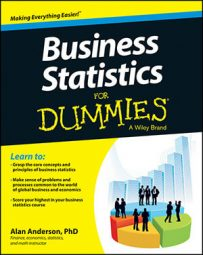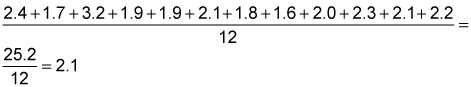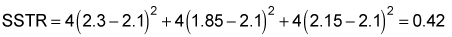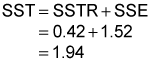Calculating the treatment sum of squares (SSTR) and the total sum of squares (SST) are two important steps in constructing the test statistic for ANOVA.

Once you have calculated the error sum of squares (SSE), you can calculate the SSTR and SST. When you compute SSE, SSTR, and SST, you then find the error mean square (MSE) and treatment mean square (MSTR), from which you can then compute the test statistic.

## How to calculate the treatment sum of squares

After you find the SSE, your next step is to compute the SSTR. This is a measure of how much variation there is among the mean lifetimes of the battery types. With a low SSTR, the mean lifetimes of the different battery types are similar to each other.

First, you need to calculate the overall average for the sample, known as the overall mean or grand mean. For example, say a manufacturer randomly chooses a sample of four Electrica batteries, four Readyforever batteries, and four Voltagenow batteries and then tests their lifetimes. This table lists the results (in hundreds of hours).

Battery Lifetimes (in Hundreds of Hours)
Battery 1 2.4 1.9 2.0
Battery 2 1.7 2.1 2.3
Battery 3 3.2 1.8 2.1
Battery 4 1.9 1.6 2.2

If you have 12 total observations (four batteries chosen from each of three battery types, as shown in the table), then you may obtain the overall mean by adding up the 12 sample values and dividing by 12:You then compute the SSTR with the following steps for each column:

1. Compute the squared difference between the column mean and the overall mean.

2. Multiply the result by the number of elements in the column.

So in this example, SSTR equalsThe calculations are based on the following results:

• There are four observations in each column.

• The overall mean is 2.1.

• The column means are 2.3 for column 1, 1.85 for column 2 and 2.15 for column 3.

After you compute SSE and SSTR, the sum of these terms is calculated, giving the SST.

## How to compute the total sum of squares

The total sum of squares (SST) equals the sum of the SSTR and the SSE. So using the battery example, you get